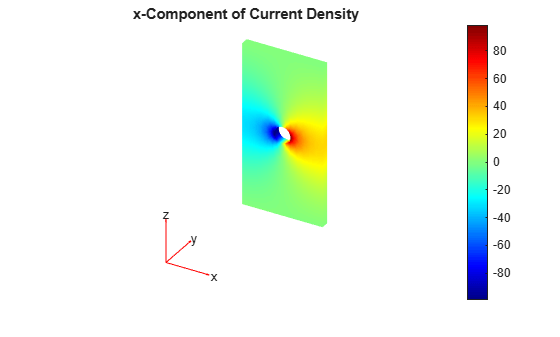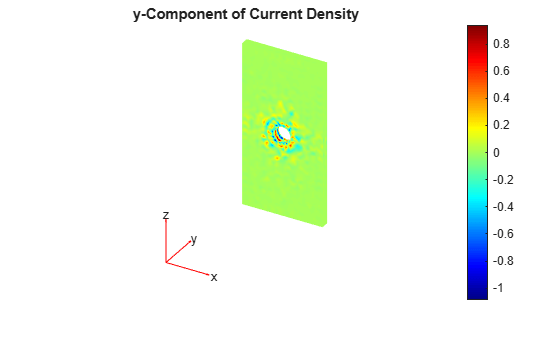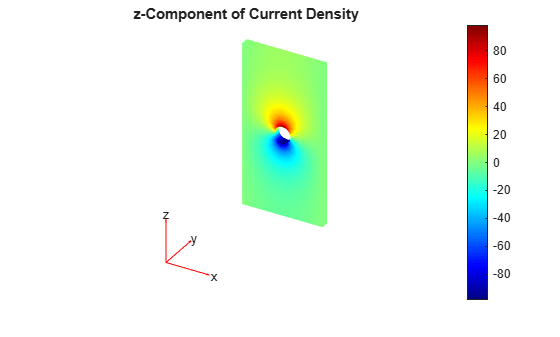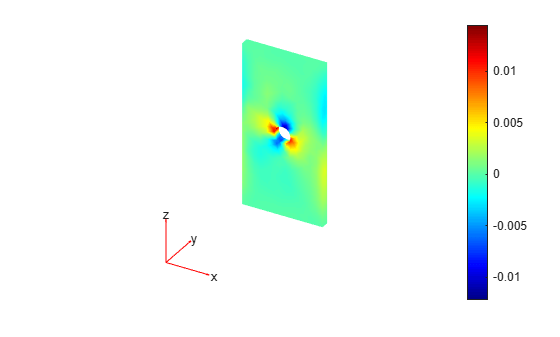# ConductionResults

DC conduction solution

## Description

A `ConductionResults` object contains the electric potential, electric field, current density, and mesh values in a form convenient for plotting and postprocessing.

The electric potential, electric field, and current density values are calculated at the nodes of the triangular or tetrahedral mesh generated by `generateMesh`. Electric potential values at the nodes appear in the `ElectricPotential` property. Electric field values at the nodes appear in the `ElectricField` property. Current density values at the nodes appear in the `CurrentDensity` property.

To interpolate the electric potential, electric field, and current density to a custom grid, such as the one specified by `meshgrid`, use the `interpolateElectricPotential`, `interpolateElectricField`, and `interpolateCurrentDensity` functions.

## Creation

Solve a DC conduction problem using the `solve` function. This function returns a solution as a `ConductionResults` object.

## Properties

expand all

Electric potential values at nodes, returned as a vector.

Data Types: `double`

Electric field values at nodes, returned as an `FEStruct` object. The properties of this object contain the components of the electric field at nodes.

Electric flux density values at nodes, returned as an `FEStruct` object. The properties of this object contain the components of the electric flux density at nodes.

Finite element mesh, returned as an `FEMesh` object. For details, see FEMesh Properties.

## Object Functions

 `interpolateElectricPotential` Interpolate electric potential in electrostatic or DC conduction result at arbitrary spatial locations `interpolateElectricField` Interpolate electric field in electrostatic or DC conduction result at arbitrary spatial locations `interpolateCurrentDensity` Interpolate current density in DC conduction result at arbitrary spatial locations

## Examples

collapse all

Solve a DC conduction problem on a geometry representing a 3-D plate with a hole in its center. Plot the electric potential and the components of the current density.

Create an electromagnetic model for DC conduction analysis.

`emagmodel = createpde("electromagnetic","conduction");`

Import and plot a geometry representing a plate with a hole.

```gm = importGeometry(emagmodel,"PlateHoleSolid.stl"); pdegplot(gm,"FaceLabels","on","FaceAlpha",0.3)```Specify the conductivity of the material.

`electromagneticProperties(emagmodel,"Conductivity",6e4);`

Apply the voltage boundary conditions on the left, right, top, and bottom faces of the plate.

`electromagneticBC(emagmodel,"Voltage",0,"Face",3:6);`

Specify the surface current density on the face bordering the hole.

`electromagneticBC(emagmodel,"SurfaceCurrentDensity",100,"Face",7);`

Generate the mesh.

`generateMesh(emagmodel);`

Solve the model.

`R = solve(emagmodel)`
```R = ConductionResults with properties: ElectricPotential: [4359x1 double] ElectricField: [1x1 FEStruct] CurrentDensity: [1x1 FEStruct] Mesh: [1x1 FEMesh] ```

Plot the electric potential.

```figure pdeplot3D(emagmodel,"ColorMapData",R.ElectricPotential)```Plot the x-component of the current density.

```figure pdeplot3D(emagmodel,"ColorMapData",R.CurrentDensity.Jx) title("x-Component of Current Density")```Plot the y-component of the current density.

```figure pdeplot3D(emagmodel,"ColorMapData",R.CurrentDensity.Jy) title("y-Component of Current Density")```Plot the z-component of the current density.

```figure pdeplot3D(emagmodel,"ColorMapData",R.CurrentDensity.Jz) title("z-Component of Current Density")```Use a solution obtained by performing a DC conduction analysis to specify current density for a magnetostatic model.

Create an electromagnetic model for DC conduction analysis.

`emagmodel = createpde("electromagnetic","conduction");`

Import and plot a geometry representing a plate with a hole.

```gm = importGeometry(emagmodel,"PlateHoleSolid.stl"); pdegplot(gm,"FaceLabels","on","FaceAlpha",0.3)```Specify the conductivity of the material.

`electromagneticProperties(emagmodel,"Conductivity",6e4);`

Apply the voltage boundary conditions on the left, right, top, and bottom faces of the plate.

`electromagneticBC(emagmodel,"Voltage",0,"Face",3:6);`

Specify the surface current density on the face bordering the hole.

`electromagneticBC(emagmodel,"SurfaceCurrentDensity",100,"Face",7);`

Generate the mesh.

`generateMesh(emagmodel);`

Solve the model.

`R = solve(emagmodel);`

Change the analysis type of the model to magnetostatic.

`emagmodel.AnalysisType = "magnetostatic";`

This model already has a quadratic mesh that you generated for the DC conduction analysis. For a 3-D magnetostatic model, the mesh must be linear. Generate a new linear mesh. The `generateMesh` function creates a linear mesh by default if the model is 3-D and magnetostatic.

`generateMesh(emagmodel);`

Specify the vacuum permeability value in the SI system of units.

`emagmodel.VacuumPermeability = 1.2566370614e-6;`

Specify the relative permeability of the material.

`electromagneticProperties(emagmodel,"RelativePermeability",5000);`

Apply the magnetic potential boundary conditions on the side faces and the face bordering the hole.

```electromagneticBC(emagmodel,"MagneticPotential",[0;0;0],"Face",3:6); electromagneticBC(emagmodel,"MagneticPotential",[0;0;0.01],"Face",7);```

Specify the current density for the entire geometry using the DC conduction solution.

`electromagneticSource(emagmodel,"CurrentDensity",R);`

Solve the model.

`Rmagnetostatic = solve(emagmodel);`

Plot the x- and z-components of the magnetic potential.

`pdeplot3D(emagmodel,"ColormapData",Rmagnetostatic.MagneticPotential.Ax)``pdeplot3D(emagmodel,"ColormapData",Rmagnetostatic.MagneticPotential.Az)`## Version History

Introduced in R2022b